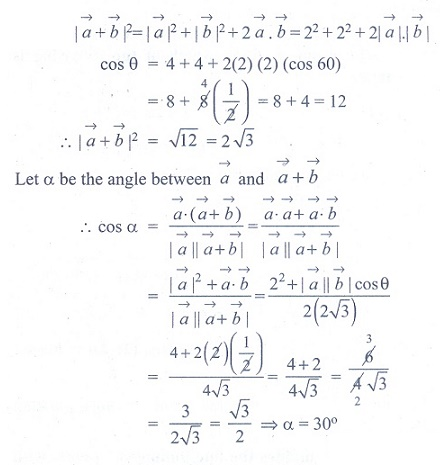# Chapter 8: Vector Algebra I - Online Test

Q1.

If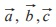are the position vectors of three collinear points, then which of the following is true?

Answer : Option B
Explaination / Solution: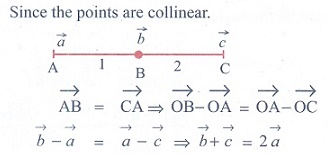Q2. If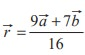, then the point P whose position vector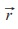divides the line joining the points with position vectorsandin the ratio
Answer : Option A
Explaination / Solution: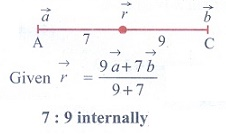Q3. If λiˆ + 2λjˆ + 2λkˆ is a unit vector, then the value of λ is
Answer : Option A
Explaination / Solution: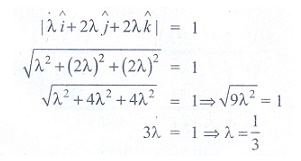Q4. Two vertices of a triangle have position vectors 3iˆ + 4 ˆj − 4kˆ and 2iˆ + 3 ˆj + 4kˆ. If the position vector of the centroid is iˆ + 2 ˆj + 3kˆ, then the position vector of the third vertex is
Answer : Option A
Explaination / Solution: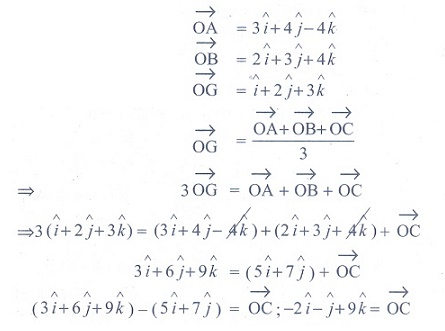Q5. If  || = 60,|| = 40 and ||=46, then || is
Answer : Option C
Explaination / Solution: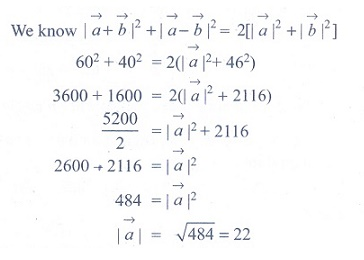Q6. Ifandhaving same magnitude and angle between them is 60º and their scalar product is 1/2 then || is
Answer : Option D
Explaination / Solution: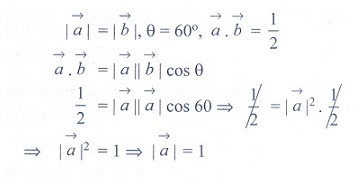Q7. The value of θ (0, π/2) for which the vectors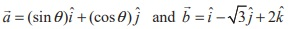are perpendicular, is equal to
Answer : Option A
Explaination / Solution: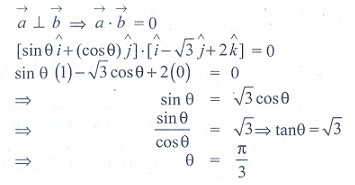Q8. If ||= 13,||= 5 and⋅= 60° then |×| is
Answer : Option D
Explaination / Solution: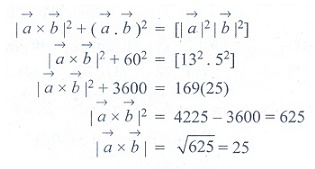Q9. Vectorsandare inclined at an angle θ = 120º. If ||= 1,||= 2 , then [(+ 3) × (−)]2  is equal to
Answer : Option D
Explaination / Solution: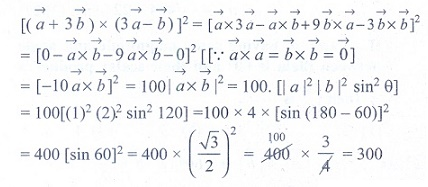Q10. Ifandare two vectors of magnitude 2 and inclined at an angle 60º , then the angle betweenand+is
Answer : Option A
Explaination / Solution: